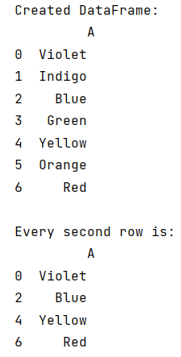# How to select every nth row in pandas?

Given a Pandas DataFrame, we have to select every nth row.
Submitted by Pranit Sharma, on June 12, 2022

## Problem statement

Given a Pandas DataFrame, you have to select and print every Nth row from it.

## Pandas Rows

Rows in pandas are the different cell (column) values which are aligned horizontally and also provides uniformity. Each row can have same or different value. Rows are generally marked with the index number but in pandas we can also assign index name according to the needs. In pandas, we can create, read, update and delete a column or row value.

## Select and print every nth Pandas row

To select every nth row of a DataFrame - we will use the slicing method. Slicing in pandas DataFrame is similar to slicing a list or a string in python. For example - if we want every 2nd row of DataFrame we will use slicing in which we will define 2 after two :: (colons).

Note:

To work with pandas, we need to import pandas package first, below is the syntax:

`import pandas as pd`

Let us understand with the help of an example,

## Python program to select every nth row in pandas

```# Importing pandas package
import pandas as pd

# Creating a dictionary
d = {'A':['Violet','Indigo','Blue','Green','Yellow','Orange','Red']}

# Create DataFrame
df = pd.DataFrame(d)

# Display DataFrame
print("Created DataFrame:\n",df,"\n")

# Selecting every 2nd row
print("Every second row is:\n",df[::2])
```

## Output

The output of the above program will be: